## RD Sharma Solutions for Class 6 Chapter 23 Data Handling - III (Bar Graphs) Free Online

Exercise 23.1 page: 23.7
1. The following table shows the daily production of T.V. sets in an industry for 7 days of a week: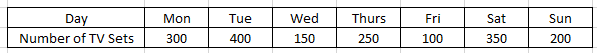Represent the above information by a pictograph.
Solution:
Consider that a TV icon represents 50 TVs.
So the number of icons produced by the industry on various days of a week are given below: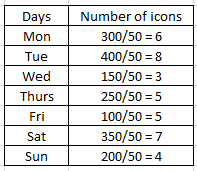Below given is the pictograph which represents the above data: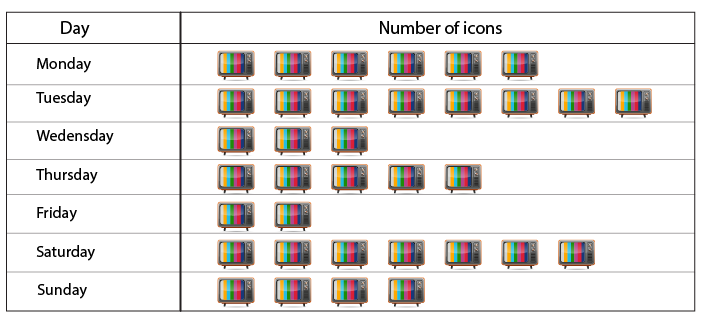2. The following table shows the number of Maruti cars sold by five dealers in a particular month: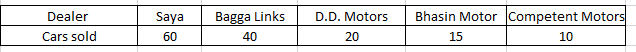Represent the above information by a pictograph.
Solution:
Consider that one car icon represents 5 Maruti cars.
So the number of icons sold by the 5 dealers in a particular month are as given below: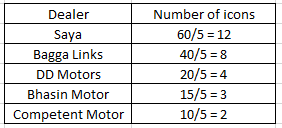Below given is the pictograph which represents the above data: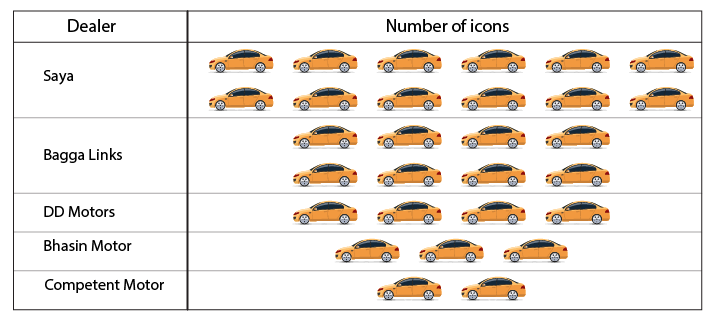3. The population of Delhi State in different census years is as given below: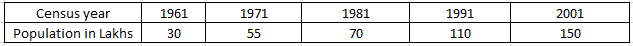Represent the above information with the help of a bar graph.
Solution:
In order to represent the data on a bar graph, we should construct a horizontal and a vertical line. We know that the horizontal line represents the census year and the vertical line represents the population in lakhs.
Here 5 values are given so mark 5 points on the horizontal axis having equal distances and erect rectangles having same width and heights proportional to the given data.
The same way, on vertical axis, difference between two points is 10 which represents a population of 10 lakhs.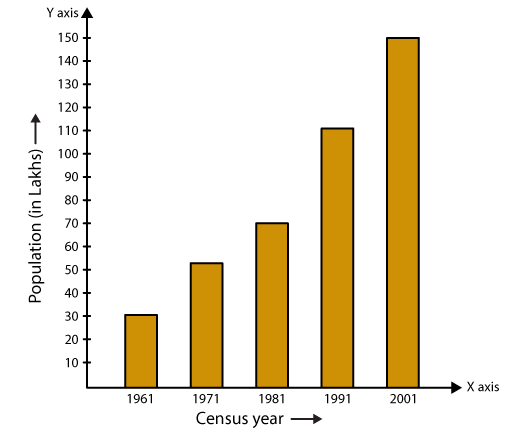4. Read the bar graph show in Fig. 23.8 and answer the following questions: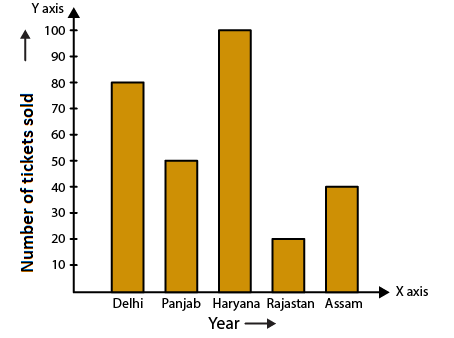(i) What is the information given by the bar graph?
(ii) How many tickets of Assam State Lottery were sold by the agent?
(iii) Of which state, were the maximum number of tickets sold?
(iv) State whether true or false.
The maximum number of tickets sold is three times the minimum number of tickets sold.
(v) Of which state were the minimum number of tickets sold?
Solution:
(i) The bar graph represents the number of tickets of different state lotteries sold by an agent on a day.
(ii) 40 tickets of Assam State Lottery were sold by the agent.
(iii) The maximum number of tickets were sold in the state Haryana.
(iv) False.
We know that
Maximum vertical length = 100 units (Haryana)
Maximum vertical length = 20 units (Rajasthan)
So the maximum number of lottery sold for one state is 100 and the minimum is 20 tickets.
(v) The minimum number of tickets were sold of Rajasthan.
5. Study the bar graph representing the number of persons in various age groups in a town shown in Fig. 23.9. Observe the bar graph and answer the following questions: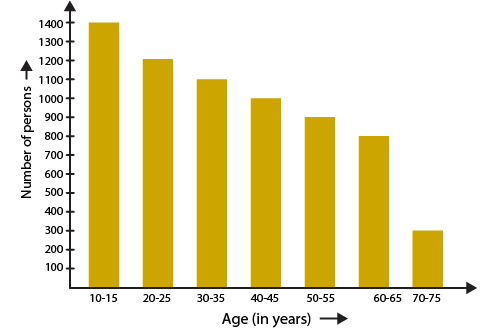(i) What is the percentage of the youngest age-group persons over those in the oldest age group?
(ii) What is the total population of the town?
(iii) What is the number of persons in the age-group 60 – 65?
(iv) How many persons are more in the age-group 10-15 than in the age group 30-35?
(v) What is the age-group of exactly 1200 persons living in the town?
(vi) What is the total number of persons living in the town in the age-group 50-55?
(vii) What is the total number of persons living in the town in the age-groups 10-15 and 60-65?
(viii) Whether the population in general increases, decreases or remains constant with the increase in the age-group.
Solution:
(i) We know that the youngest age is 10-15 years.
No. of persons in the youngest age group = 1400
70-75 years is the oldest age group.
No. of persons in the oldest age group = 300
So the difference = 1400 – 300 = 1100
Hence, the youngest group has 1100 more people than the oldest group.
Percentage of the youngest group over oldest group = (1100/300) × 100 = 1100/3 = 366 2/3 %
(ii) We know that the total population of the town = total number of people from all age groups
By substituting the values
Total population of the town = 1400 + 1200 + 1100 + 1000 + 900 + 800 + 300 = 6700
(iii) From the bar graph we know that the age group 60-65 years consists of 800 persons.
(iv) No. of persons in the age group 10-15 = 1400
No. of persons in the age group 30-35 = 1100
So the number of more persons in the age group 10-15 when compared to that of 30-35 = 1400 – 1100 = 300
(v) We know that 1200 people are living in the age group 20-25 years.
(vi) No. of people of the age group 50-55 is 900.
(vii) We know that 1400 persons exist of the age group 10-15 years and 9800 exist in the age group 60-65 years.
So the total number of persons in the age group 10-15 years and 60-65 years = 1400 + 800 = 2200
(viii) We know that increase in the age group has decrease in population.
6. Read the bar graph shown in Fig. 23.10 and answer the following questions:
(i) What is the information given by the bar graph?
(ii) What was the number of commercial banks in 1977?
(iii) What is the ratio of the number of commercial banks in 1969 to that in 1980?
(iv) State whether true or false:
The number of commercial banks in 1983 is less than double the number of commercial banks in 1969.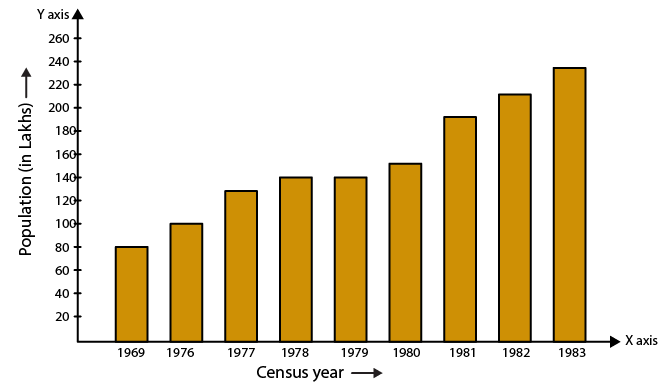Solution:
(i) The bar graph represents the number of commercial banks in India during some years.
(ii) 130 was the number of commercial banks in 1977.
(iii) No. of commercial banks in 1969 = 90
No. of commercial banks in 1980 = 150
Hence, the ratio of the number of commercial banks in 1969 to that in 1980 = 90/150 = 3/5 = 3: 5.
(iv) False.
We know that
No. of commercial banks in 1983 = 230
No. of commercial banks in 1969 = 90
So we get 2 × 90 = 180
Here, 230 is greater than 180 which means the number of commercial banks in 1983 is not less than double the number of commercial banks in 1969.
7. Given below (Fig. 23.11) is the bar graph indicating the marks obtained out of 50 in mathematics paper by 100 students. Read the bar graph and answer the following questions: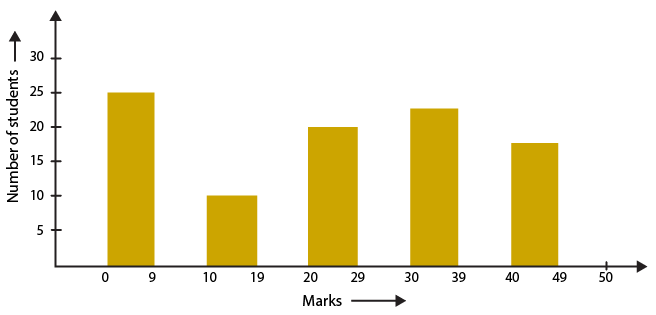(i) It is decided to distribute work books on mathematics to the students obtaining less than 20 marks, giving one workbook to each of such students. If a work book costs Rs. 5, what sum is required to buy the work books?
(ii) Every student belonging to the highest mark group is entitled to get a prize of Rs. 10. How much amount of money is required for distributing the prize money?
(iii) Every student belonging to the lowest mark-group has to solve 5 problems per day. How many problems, in all, will be solved by the students of this group per day?
(iv) State whether true or false.
(a) 17% students have obtained marks ranging from 40 to 49.
(b) 59 students have obtained marks ranging from 10 to 29.
(v) What is the number of students getting less than 20 marks?
(vi) What is the number of students getting more than 29 marks?
(vii) What is the number of students getting marks between 9 and 40?
(viii) What is the number of students belonging to the highest mark group?
(ix) What is the number of students obtaining more than 19 marks?
Solution:
Below given is the chart of 100 students by using the information from the bar graph.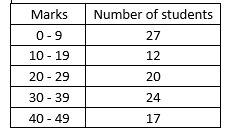(i) No. of students who score less than 20 marks = 27 + 12 = 39
Amount required to buy the workbooks = 5 × 39 = Rs 195
(ii) We know that the highest marks group is 40 – 49
No. of students in this marks group = 17
Money required to distribute the prize = 10 × 17 = Rs 170
(iii) We know that the lowest marks group is 0 – 9
No. of students in this marks group = 27
No. of problems that will be solved by students each day = 5 × 27 = 135
(iv) (a) True
(b) False
(v) No. of students scoring less than 20 marks = No. of students in 0-9 marks group + No. of students in 10-19 marks group
By substituting the values
No. of students scoring less than 20 marks = 27 + 12 = 39
(vi) No. of students scoring more than 29 marks = No. of students in 30-39 marks group + No. of students in 40-49 marks group
By substituting the values
No. of students scoring more than 29 marks = 24 + 17 = 41
(vii) No. of students who score between 9 and 40 = No. of students in 10-19 marks group + No. of students in 20-29 marks group + No. of students in 30-39 marks group
By substituting the values
No. of students who score between 9 and 40 = 12 + 30 + 24 = 56
(viii) We know that 40-49 is the highest marks group
So the number of students in this group is 17.
(ix) No. of students who score more than 19 marks = No. of students in 20-29 marks group + No. of students in 30-39 marks group + No. of students in 40-49 marks group
By substituting the values
No. of students who score more than 19 marks = 20 + 24 + 17 = 61
8. Read the following bar graph (Fig. 23.12) and answer the following questions: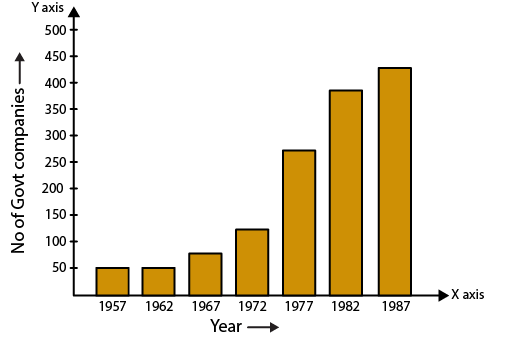(i) What is the information given by the bar graph?
(ii) State each of the following whether true or false.
(a) The number of government companies in 1957 is that of 1982 is 1: 9.
(b) The number of government companies have decreased over the year 1957 to 1983.
Solution:
(i) The bar graph represents the number of government companies in India during some years.
(ii) (a) False.
We know that
No. of government companies in 1957 = 50
No. of government companies in 1982 = 375
So the ratio = 50/375 = 2/15 = 2: 15
(b) False. There is no data given for 1983. Hence the statement is not true.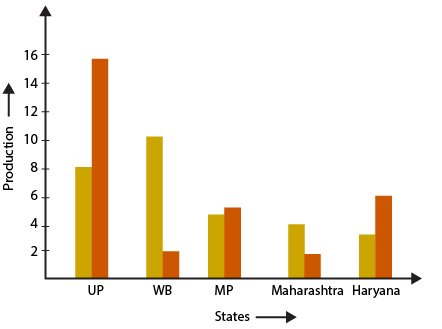(i) What information is given by the bar graph?
(ii) Which state is the largest producer of rice?
(iii) Which state is the largest producer of wheat?
(iv) Which state has total production of rice and wheat as its maximum?
(v) Which state has the total production of wheat and rice minimum?
Solution:
Consider a chart by using data from the bar graph: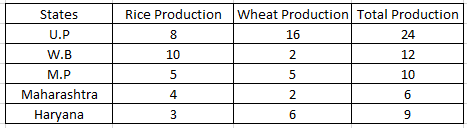(i) The bar graph gives information regarding rice and wheat production in various states.
(ii) The largest producer of rice is W.B.
(iii) The largest producer of wheat is U.P.
(iv) U.P has the total production of rice and wheat as its maximum.
(v) Maharashtra has the total production of wheat and rice minimum.
10. The following bar graph (Fig. 23.14) represents the heights (in cm) of 50 students of Class XI of a particular school. Study the graph and answer the following questions: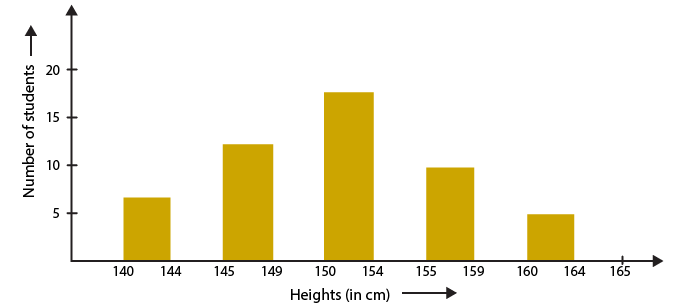(i) What percentage of the total number of student have their heights more than 149 cm?
(ii) How many students in the class are in the range of maximum height of the class?
(iii) The school wants to provide a particular type of tonic to each student below the height of 150 cm to improve his height. If the cost of the tonic for each student comes out to be Rs 55, how much amount of money is requires?
(iv) How many students are in the range of shortest height of the class?
(v) State whether true or false:
(a) There are 9 students in the class whose heights are in the range of 155−159 cm.
(b) Maximum height (in cm) of a student in the class is 17.
(c) There are 29 students in the class whose heights are in the range of 145−154 cm.
(d) Minimum height (in cm) of a student is the class is in the range of 140−144 cm.
(e) There are 14 students each of whom has height more than 150 cm is 12.
(f) There are 14 students each of whom has height more than 154 cm.
Solution:
Consider a chart by using data from the bar graph: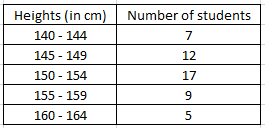(i) No. of students having height more than 149 cm = 17 + 9 + 5 = 31
Total students = 50
So the percentage of students having height more than 149 cm = 31/50 = 31 × 2 = 62%
(ii) Maximum height range of the class is 160-164
No. of students in this range = 5
(iii) No. of students having height less than 150 cm = 7 + 12 = 19
Amount required to be spent for the tonic = 19 × 55 = Rs 1045
(iv) We know that minimum height range of the class is 140-144
No. of students in this range = 7
(v) (a) True. No. of students in the height range 155 – 159 is 9.
(b) False. No. of students in the maximum height range 160 – 164 is 17.
(c) True.
No. of students having heights in the range 145 – 154 cm = No. of students having heights in the range 145-149 + No. of students having heights in the range 150-154
We get
No. of students having heights in the range 145 – 154 cm = 12 + 17 = 29
(d) True. 140-144 cm is the minimum height range of the students.
(e) False.
No. of students having heights less than 150 cm = 7 + 12 = 19
(f) True.
No. of students having heights more than 154 cm = 9 + 5 = 14
11. Read the following bar graph (Fig.23.15) and answer the following questions: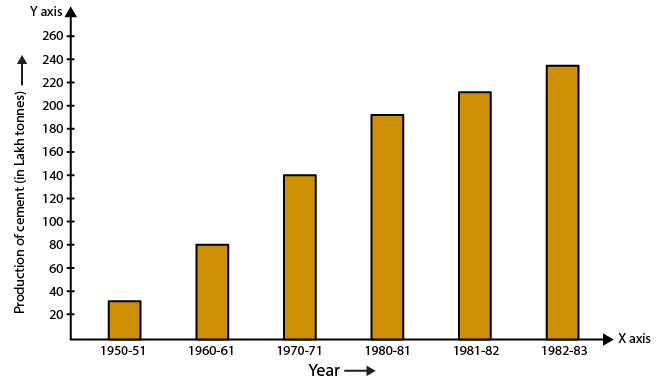(i) What information is given by the bar graph?
(ii) What was the production of cement in the year 1980-81?
(iii) What is the minimum and maximum productions of cement and corresponding years?
Solution:
(i) The bar graph represents industrial production of cement in different years in India.
(ii) The production of cement in the year 1980-81 is 186 lakh tonnes.
(iii) We know that minimum height of bar in 1950-51 is 30 units.
Hence, minimum production is 30 lakh tonnes in 1950-51.
We know that maximum height of bar in 1982-83 is 232 units.
Hence, maximum production is 232 lakh tonnes in 1982-83.
12. The bar graph shown in Fig. 23.16 represents the circulation of newspapers in 10 languages. Study the bar graph and answer the following questions: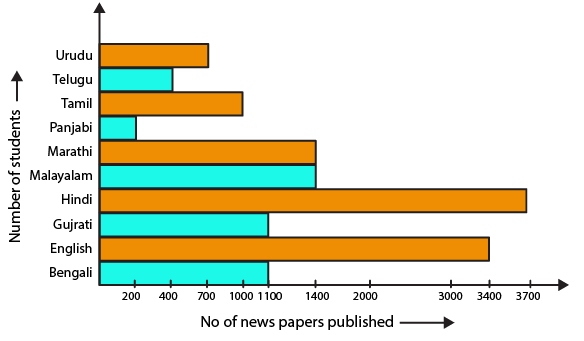(i) What is the total number of newspapers published in Hindi, English, Urdu, Punjabi and Bengali?
(ii) What percent is the number of newspapers published in Hindi of the total number of newspapers?
(iii) Find the excess of the number of newspapers, published in English over those published in Urdu.
(iv) Name two pairs of languages which publish the same number of newspapers.
(v) State the language in which the smallest number of newspapers are published.
(vi) State the language in which the number of newspapers are published.
(vii) State the language in which the number of newspapers published is between 2500 and 3500.
(viii) State whether true or false:
(a) The number of newspapers published in Malayalam and Marathi together is less than those published in English.
(b) The number of newspapers published in Telugu is more than those published in Tamil.
Solution:
Consider a chart by using data from the bar graph: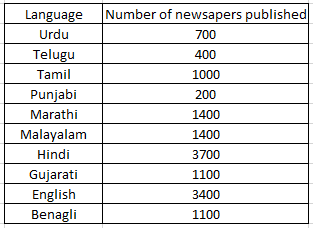(i) We know that the total number of newspapers published = 3700 + 3400 + 700 + 200 + 1100 = 9100
(ii) No. of newspapers published in Hindi = 3700
Total newspapers published = 700 + 400 + 1000 + 200 + 1400 + 1400 + 3700 + 1100 + 3400 + 1100 = 14400
% of Hindi newspaper published = 3700/14400 × 100 = 25.7%
(iii) No. of English newspapers published = 3400
No. of Urdu newspapers published = 700
So the excess number of newspapers which are published in English over Urdu = 3400 – 700 = 2700
(iv) Gujarati and Bengali, Marathi and Malayalam are the two pairs in which same number of newspapers are published.
(v) The smallest number of newspapers are published in Punjabi language.
(vi) The maximum number of newspapers are published in Hindi language.
(vii) The number of newspapers published is between 2500 and 3500 in English language.
(viii) (a) True.
No. of newspapers in Malayalam and Marathi = 1400 + 1400 = 2800
No. of English newspapers is 3400 which is more than total number of Malayalam and Marathi newspapers.
(b) False.
No. of newspapers published in Telugu = 400
No. of newspapers published in Tamil = 1000

Exercise 23.2 page: 23.18
1. Explain the reading and interpretation of bar graphs.
Solution:
A graph with its length proportional to the value it represents is called a bar graph. The bars can either be [plotted horizontally or vertically. It is basically a visual display which is used to compare the amount of occurrence of carious characteristics of data.
Bar graph help us to
(i) Compare the groups of data
(ii) Generalize the data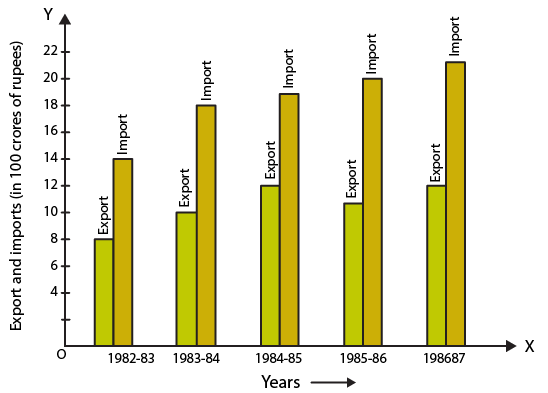(i) What information is given by the bar graph?
(ii) In which year the export is minimum?
(iii) In which year the import is maximum?
(iv) In which year the difference of the values of export and import is maximum?
Solution:
Consider a chart by using data from the bar graph: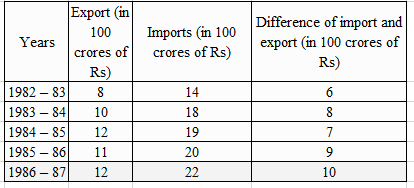(i) The bar graph gives us information regarding import and export from 1982-83 to 1886-87.
(ii) The export is minimum in the year 1982-83.
(iii) The import is maximum in the year 1986-87.
(iv) The difference of the values of import and export is maximum in the year 1986-87.
3. The following bar graph shows the results of an annual examination in a secondary school.
Read the bar graph (Fig.23.22) and choose the correct option in each of the following: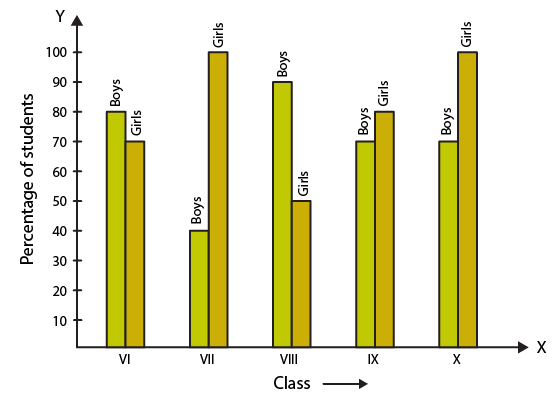(i) The pair of classes in which the results of boys and girls are inversely proportional are:
(a) VI, VIII
(b) VI, IX
(c) VIII, IX
(d) VIII, X
(ii) The class having the lowest failure rate of girls is:
(a) VII
(b) X
(c) IX
(d) VIII
(iii) The class having the lowest pass rate of student is:
(a) VI
(b) VII
(c) VIII
(d) IX
Solution:
Consider a chart by using data from the bar graph: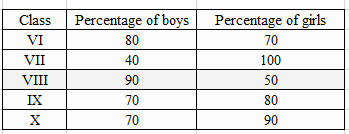(i) The option (b) is the correct answer.
The pair of classes in which the results of boys and girls are inversely proportional are VI, IX.
We know that
In class VI
% of boys = 80
% of girls = 70
In class IX
% of boys = 80
% of girls = 70
(ii) The option (a) is the correct answer.
The class having the lowest failure rate of girls is VII.
We know that the passing percentage of girls in Class VII is 100%.
So 0% of girls have failed in this is class.
(iii) The option (b) is the correct answer.
The class having the lowest pass rate of student is VII.
We know that the sum of vertical heights of % of boys and girls in class VII is same i.e. 140 units and the sum is least compared to other classes.
4. The following bar graph shows the number of persons killed in industrial accidents in a country for some years (Fig.23.23).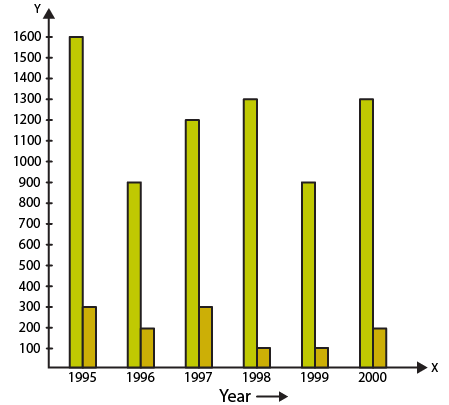Read the bar graph and choose the correct alternative in each of the following:
(i) The year which shows the maximum percentage increase in the number of persons killed in coal mines over the preceding year is:
(a) 1996
(b) 1997
(c) 1999
(d) 2000
(ii) The year which shows the maximum decrease in the number of persons killed in industrial accidents over the preceding year is:
(a) 1996
(b) 1997
(c) 1998
(d) 1999
(iii) The year in which the maximum number of persons were killed in industrial accidents other than those killed in coal mines is:
(a) 1995
(b) 1997
(c) 1998
(d) 1999
Solution:
Consider a chart by using data from the bar graph: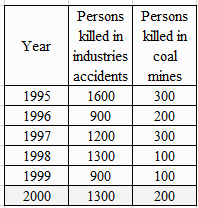(i) The option (d) is the correct answer.
The year which shows the maximum percentage increase in the number of persons killed in coal mines over the preceding year is 2000.
In the year 1997 the death increased to 300 from 200 and in the year 200 increased to 200 from 100.
% increase in death in 1997 = 50%
% increase in death in 2000 = 100%
(ii) The option (a) is the correct answer.
The year which shows the maximum decrease in the number of persons killed in industrial accidents over the preceding year is 1996.
The year 1996 and 1999 show decrease in the amount of persons killed by industrial accidents.
% decrease in the death in 1996 = 43.75%
% decrease in the death in 1999 = 30.77%
(iii) The option (a) is the correct answer.
The year in which the maximum number of persons were killed in industrial accidents other than those killed in coal mines is 1995.
1600 persons were killed in the year 1995 due to industrial accidents which is higher when compared to other years.
5. The production of saleable steel in some of the steel plants of our country during 1999 is given below: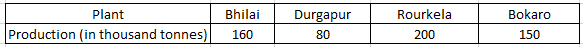Construct a bar graph to represent the above data on a graph paper by using the scale 1 big divisions = 20 thousand tonnes.
Solution:
Construct two mutually perpendicular lines OX and OY.
Let us mark plants along the horizontal line OX and mark production along the vertical line OY.
Take equal width for each bar on the axis OX.
Now let us take a suitable scale to find the heights of the bar.
Take 1 division = 20 thousand tons
So the heights of the bars are as given below:
Bhilai = 160/20 = 8 units
Durgapur = 80/20 = 4 units
Rourkela = 200/20 = 10 units
Bokaro = 150/20 = 7.5 units
Using the above calculation, the graph is as given below: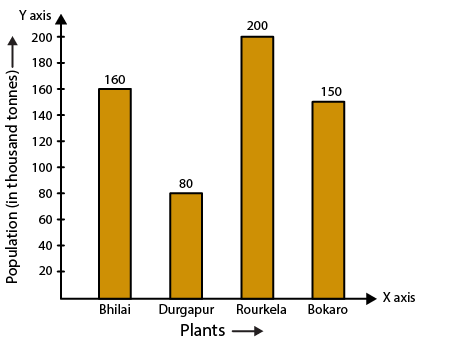6. The following data gives the number (in thousands) of applicants registered with an Employment Exchange during. 1995-2000: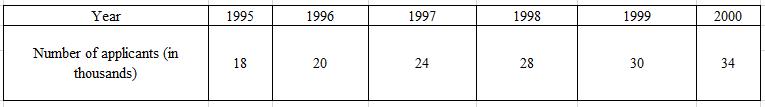Construct a bar graph to represent the above data.
Solution:
Construct two mutually perpendicular lines OX and OY.
Let us mark years along the horizontal line OX and mark number of applicants registered along the vertical line OY.
Take equal width for each bar on the axis OX.
Now let us take a suitable scale to find the heights of the bar.
Take 1 division = 4 thousand applicants
So the heights of the bars are as given below:
1995 = 18/4 = 4.5 units
1996 = 20/4 = 5 units
1997 = 24/4 = 6 units
1998 = 28/4 = 7 units
2000 = 34/4 = 8.5 units
Using the above calculation, the graph is as given below: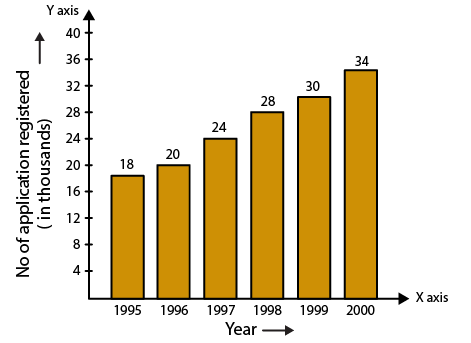7. The following table gives the route length (in thousand kilometres) of the Indian Railways in some of the years: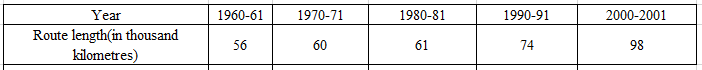Represent the above data with the help of a bar graph.
Solution:
Construct two mutually perpendicular lines OX and OY.
Let us mark years along the horizontal line OX and mark route length along the vertical line OY.
Take equal width for each bar on the axis OX.
Now let us take a suitable scale to find the heights of the bar.
Take 1 big division = 10 thousand kilometres
So the heights of the bars are as given below:
1960-61 = 56/10 = 5.6 units
1970-71 = 60/10 = 6 units
1980-81 = 61/10 = 6.1 units
1990-91 = 74/10 = 7.4 units
2000-2001 = 98/10 = 9.8 units
Using the above calculation, the graph is as given below: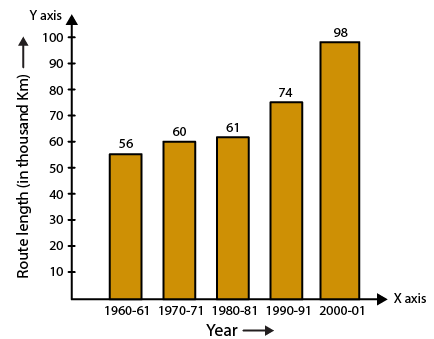8. The following data gives the amount of loans (in crores of rupees) disbursed by a bank during some years: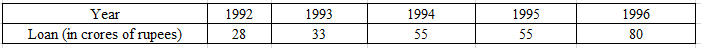(i) Represent the above data with the help of a bar graph.
(ii) With the help of the bar graph, indicate the year in which amount of loan is not increased over that of the preceding year.
Solution:
(i) Construct two mutually perpendicular lines OX and OY.
Let us mark years along the horizontal line OX and mark loans in crores along the vertical line OY.
Take equal width for each bar on the axis OX.
Now let us take a suitable scale to find the heights of the bar.
Take 1 big division = 10 crores of loan
So the heights of the bars are as given below:
1992 = 28/10 = 2.8 units
1993 = 33/10 = 3.3 units
1994 = 55/10 = 5.5 units
1995 = 55/10 = 5.5 units
1996 = 80/10 = 8.0 units
Using the above calculation, the graph is as given below: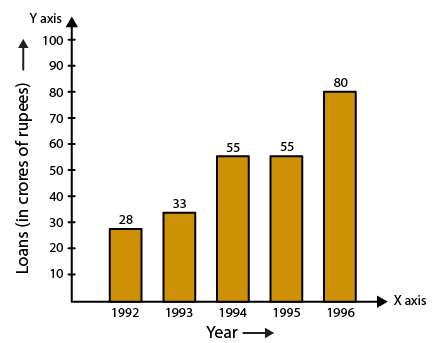(ii) 1995 is the year where the loan amount has not increased than its previous year.
9. The following table shows the interest paid by a company (in lakhs):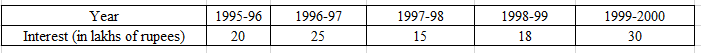Draw the bar graph to represent the above information.
Solution:
Construct two mutually perpendicular lines OX and OY.
Let us mark years along the horizontal line OX and mark amount of interest paid by the company along the vertical line OY.
Take equal width for each bar on the axis OX.
Now let us take a suitable scale to find the heights of the bar.
Take 1 big division = 5 lakhs of rupees paid as interest by the company
So the heights of the bars are as given below:
1995-96 = 20/5 = 4 units
1996-97 = 25/5 = 5 units
1997-98 = 15/5 = 3 units
1998-99 = 18/5 = 3.6 units
1999-2000 = 30/5 = 6 units
Using the above calculation, the graph is as given below: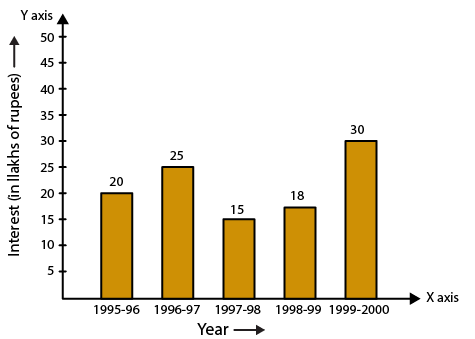10. The following data shows the average age of men in various countries in a certain year: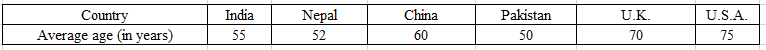Represent the above information by a bar graph.
Solution:
Construct two mutually perpendicular lines OX and OY.
Let us mark countries along the horizontal line OX and mark average age for men along the vertical line OY.
Take equal width for each bar on the axis OX.
Now let us take a suitable scale to find the heights of the bar.
Take 1 big division = 10 years
So the heights of the bars are as given below:
India = 55/10 = 5.5 units
Nepal = 52/10 = 5.2 units
China = 60/10 = 6.0 units
Pakistan = 50/10 = 5.0 units
UK = 70/10 = 7 units
USA = 75/10 = 7.5 units
Using the above calculation, the graph is as given below: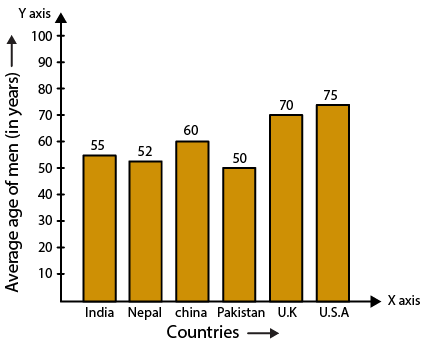11. The following data gives the production of foodgrains (in thousand tonnes) for some years: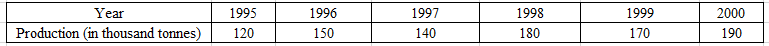Represent the above data with the help of a bar graph.
Solution:
Construct two mutually perpendicular lines OX and OY.
Let us mark years along the horizontal line OX and mark production of food grains in tonnes along the vertical line OY.
Take equal width for each bar on the axis OX.
Now let us take a suitable scale to find the heights of the bar.
Take 1 big division = 20 thousand tonnes
So the heights of the bars are as given below:
1995 = 120/20 = 6 units
1996 = 150/20 = 7.5 units
1997 = 140/20 = 7 units
1998 = 180/20 = 9 units
1999 = 170/20 = 8.0 units
2000 = 190/20 = 9.5 units
Using the above calculation, the graph is as given below: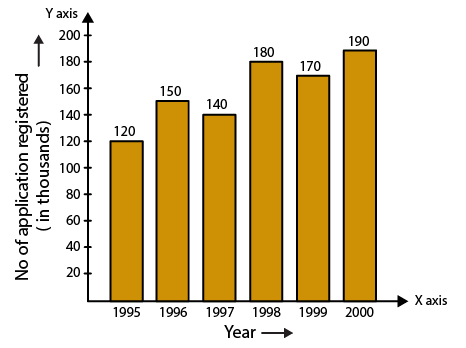12. The following data gives the amount of manure (in thousand tonnes) manufactured by a company during some years: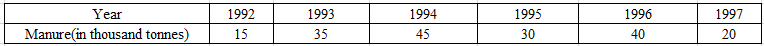(i) Represent the above data with the help of a bar graph.
(ii) Indicate with the help of the bar graph the year in which the amount of manure manufactured by the company was maximum.
(iii) Choose the correct alternative:
The consecutive years during which there was maximum decrease in manure production are:
(a) 1994 and 1995
(b) 1992 and 1993
(c) 1996 and 1997
(d) 1995 and 1996
Solution:
(i) Construct two mutually perpendicular lines OX and OY.
Let us mark years along the horizontal line OX and mark amount of manure in tonnes along the vertical line OY.
Take equal width for each bar on the axis OX.
Now let us take a suitable scale to find the heights of the bar.
Take 1 big division = 5 thousand tonnes of manure
So the heights of the bars are as given below:
1992 = 15/5 = 3 units
1993 = 35/5 = 7 units
1994 = 45/5 = 9 units
1995 = 30/5 = 6 units
1996 = 40/5 = 8.0 units
1997 = 20/5 = 4 units
Using the above calculation, the graph is as given below: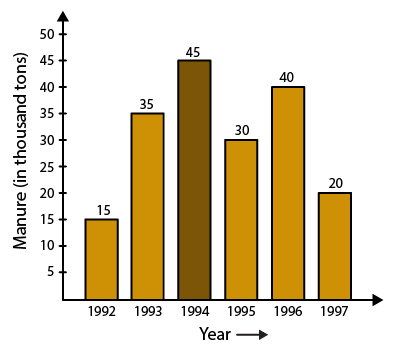(ii) Maximum amount of manure was manufactured by the company in the year 1994.
(iii) The option (c) is the correct answer.
The consecutive years during which there was maximum decrease in manure production are 1996 and 1997.

Production in the year 1996 and 1997 was decreased by 20 thousand tonnes.
Courtesy : CBSE